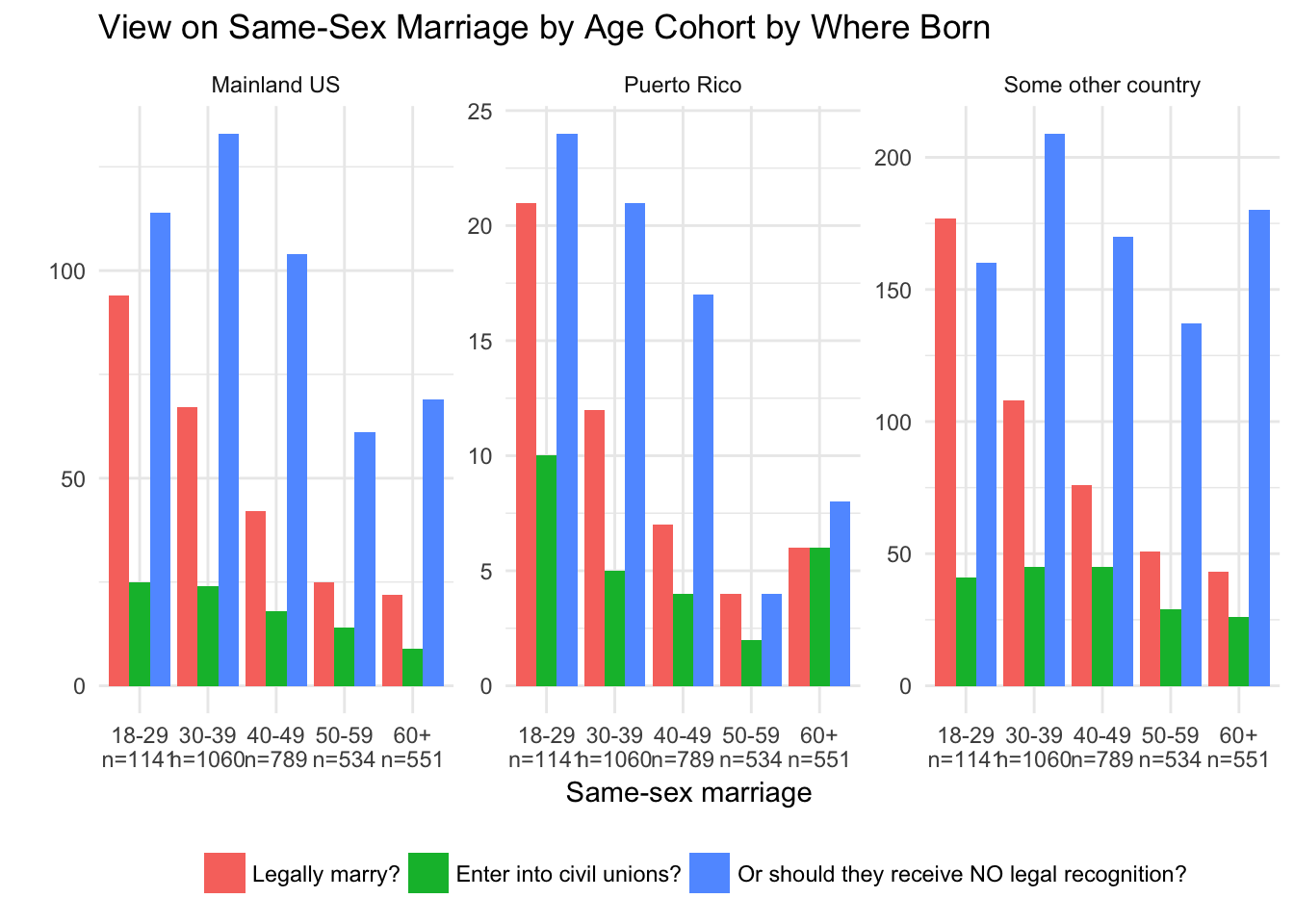1. Open RStudio
2. Go to File: New File: RScript
• The type of file you have just opened, a .R file, is nothing more than a regular text file. You can open it in Microsoft Word, or a text editor, or whatever. We’re creating and working with it in RStudio because RStudio talks to the programming language .R. You will only ever practically use a .R file in RStudio.
3. At the top of you .R script, type two pound signs or, as the kids call it these days, a ‘hashtag,’ followed by your name, and then again on another line for the date, and then again on another line for the phrase ‘US LATINO POLITICS’ like so.
## John Ray
## Whatever today is
## US LATINO POLITICS
1. Save obsessively.
2. To read in the Stata dataset we will use the function read.dta from the foreign library. A ‘library’ is just a bundle of functions that expands the functionality of your R setup, like installing an add-on to your browser or putting moon shoes on a cat. You only have to install a library once to use it, so run the line of code install.packages('foreign') then delete it. To load the library once you’ve installed it, run library(foreign). So the entirety of your script so far should look like
## John Ray
## Whatever today is
## US LATINO POLITICS

library(foreign)
1. Lets load the dataset, which we will store in a variable called dat.
dat <- read.dta('http://mattbarreto.com/mbarreto/courses/LNS_dataset.dta')

If it worked, you should get some wacky warning message you can ignore that looks like this: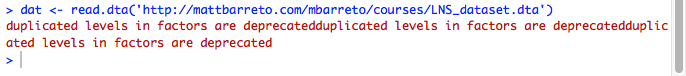and you should see a new variable in your global environment: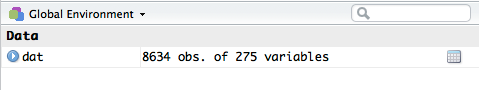Save obsessively.

library(gmodels)
table(dat$LANGPREF) ## ## English Spanish ## 3291 5343 The output here shows that, of the survey’s 8,634 respondents, 3,291 respondents said their language preference was English, and 5,343 respondents said their language preference was Spanish. We can also make a table of two variables. table(dat$LANGPREF, dat$IDPREF) ## ## Hispanic Latino Either is acceptable Don't care DK/NA ## English 1148 434 698 963 0 ## Spanish 1882 681 2119 602 0 A table of two or more variables is called a cross-tab (cross table). To say the line of code above out loud, you would say, I’m making a cross-tab of age by idpref.’’ Here, we see that 1,148 resopndents said they preferred English and also that they prefer to identify as Hispanic, 434 respondents said they preferred English and also that they prefer to identify as Latino, 1,882 prefer English and identy as Hispanic, and so on. And if you really want a rush, you can make a table of three variables. table(dat$LANGPREF, dat$IDPREF, dat$BORNUS)
## , ,  = Mainland US
##
##
##           Hispanic Latino Either is acceptable Don't care DK/NA
##   English      791    255                  421        585     0
##   Spanish      124     62                  131         49     0
##
## , ,  = Puerto Rico
##
##
##           Hispanic Latino Either is acceptable Don't care DK/NA
##   English       67     39                   45         68     0
##   Spanish       80     27                  106         26     0
##
## , ,  = Some other country
##
##
##           Hispanic Latino Either is acceptable Don't care DK/NA
##   English      290    140                  232        310     0
##   Spanish     1678    592                 1882        527     0

Holy moley.

This is getting to be a bit much. It’d be even more so if you tried to produce a table of a variable with LOTS of values like, say, the age variable (dat$AGE). If you’re working with a variable like age, you’ll probably want to recode it into bins, for simplicity’s sake. Lets say I want to recode the AGE variable so that the people 18-29 are in bin 1, people in 30-39 in bin 2, 40-49 in bin 3, 50-59 in bin 4, and 60+ in bin 5. We will store this recode in a new variable called AGE_CAT. dat$AGE_CAT <- dat$AGE dat$AGE_CAT[dat$AGE %in% c(18:29)] <- 1 dat$AGE_CAT[dat$AGE %in% c(30:39)] <- 2 dat$AGE_CAT[dat$AGE %in% c(40:49)] <- 3 dat$AGE_CAT[dat$AGE %in% c(50:59)] <- 4 dat$AGE_CAT[dat$AGE %in% c(60:100)] <- 5 table(dat$AGE_CAT)
##
##    1    2    3    4    5
## 2299 2061 1591 1096 1094

From here, the easiest way to transfer your tabular data into a chart is probably to just copy and paste it into Excel, and pretty it up that way. I have heard that making charts with Excel these days is pretty easy these days, and if thats what works best for you, go for it.

But if you really wanna bump up your nerd street cred, you’ll want to make your plot in R using its plotting library, ggplot2. If you want to try that, first install ggplot2 by running install.packages(ggplot2) and then adding a line of code to your .R file to load it:

library(ggplot2)

Remember you’ve got a dataframe, dat and inside it are variables you access using the $ operator, like dat$AGE to get the age variable, and so on. In the following section, I’ve written some code that’ll make your plots relatively easy. Copy and paste those into your .R file and run them without changing them.

plot_2_variables = function(dataframe = NA, x_variable = NA, y_variable = NA, x_variable_label = 'X variable label', y_variable_label = 'Y variable label', x_axis_tick_labels = NA, bin_n = NA, legend_title = NA, plot_title = NA, x_responses_to_ignore = NA, y_responses_to_ignore = NA){
pdat <- dataframe[complete.cases(dataframe[,c(x_variable, y_variable)]),]
pdat <- pdat[!as.character(pdat[,x_variable]) %in% x_responses_to_ignore & !as.character(pdat[,y_variable]) %in% y_responses_to_ignore,]

break_seq <- 1:length(unique(pdat[,x_variable]))
x_tick_labs <- paste0(x_axis_tick_labels,'\n(n = ',bin_n,')')

gg <- ggplot(pdat, aes(x = factor(pdat[,x_variable]), fill = pdat[,y_variable], label = pdat[,y_variable])) +
labs(x = x_variable_label, y = y_variable_label, title = plot_title) +
scale_x_discrete(breaks = break_seq, label = x_tick_labs) +
guides(fill = guide_legend(title = legend_title)) +
geom_bar(position = 'dodge') +
theme_minimal() +
theme(legend.position = 'bottom')

return(gg)
}

plot_3_variables = function(dataframe = NA, x_variable = NA, y_variable = NA, z_variable = NA, x_variable_label = 'X variable label', y_variable_label = 'Y variable label', x_axis_tick_labels = NA, bin_n = NA, legend_title = NA, plot_title = NA, x_responses_to_ignore = NA, y_responses_to_ignore = NA, z_responses_to_ignore = NA, ncol = 10){
pdat <- dataframe[complete.cases(dataframe[,c(x_variable, y_variable)]),]
pdat <- pdat[!as.character(pdat[,x_variable]) %in% x_responses_to_ignore & !as.character(pdat[,y_variable]) %in% y_responses_to_ignore & !as.character(pdat[,z_variable]) %in% z_responses_to_ignore,]

break_seq <- 1:length(unique(pdat[,x_variable]))
x_tick_labs <- paste0(x_axis_tick_labels,'\nn=',bin_n,'')

gg <- ggplot(pdat, aes(x = factor(pdat[,x_variable]), fill = pdat[,y_variable], label = pdat[,y_variable])) +
labs(x = x_variable_label, y = y_variable_label, title = plot_title) +
scale_x_discrete(breaks = break_seq, label = x_tick_labs) +
guides(fill = guide_legend(title = legend_title)) +
geom_bar(position = 'dodge') +
theme_minimal() +
theme(legend.position = 'bottom') +
facet_wrap(~pdat[,z_variable], ncol = ncol, scales = "free")

return(gg)
}

Once you’ve done that, you should see two new objects in your global environment under the Functions’’ area, like so: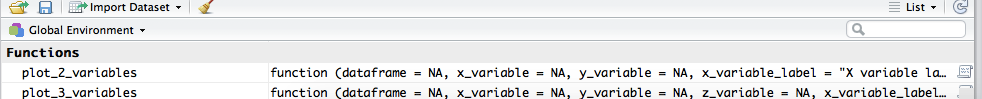To use this code, on a new line (not in the original lines where you defined the functions plot_2_variables and plot_3_variables!) type plot_2_variables() and, in the parentheses, fill in the following arguments:

• dataframe: The name of your dataframe, probably dat
• x_variable: The name of your first variable with quotes on either side, like ‘AGE_CAT’ for AGE_CAT
• y_variable: The name of your second variable, also with quotes
• x_variable_label: A label for your x variable, also with quotes
• y_variable_label: A label for your y variable, w quotes
• x_axis_tick_labels: A vector of labels for your tick marks, one label for each unique response to the survey question
• bin_n: The number of respondents in each bin, which you get from running table
• legend_title: The title of the legend, in quotes
• plot_title: The plot title, in quotes
• x_responses_to_ignore: Responses to ignore in your first variable, usually for NAs
• y_responses_to_ignore: Responses to ignore in your second variable, usually for NAs

For example, here is how to use the function if your variables of interest are AGE_CAT and SAMESEX:

plot_2_variables(
dataframe = dat,
x_variable = 'AGE_CAT',
y_variable = 'SAMESEX',
x_variable_label = 'Same-sex marriage',
y_variable_label = '',
x_axis_tick_labels = c('18 to 29', '30 to 39', '40 to 49', '50 to 59', '60 or older'),
bin_n = c(1141, 1060, 789, 534, 551),
legend_title = '',
plot_title = 'View on Same-Sex Marriage by Age Cohort',
x_responses_to_ignore = NA,
y_responses_to_ignore = 'No opinion/NA')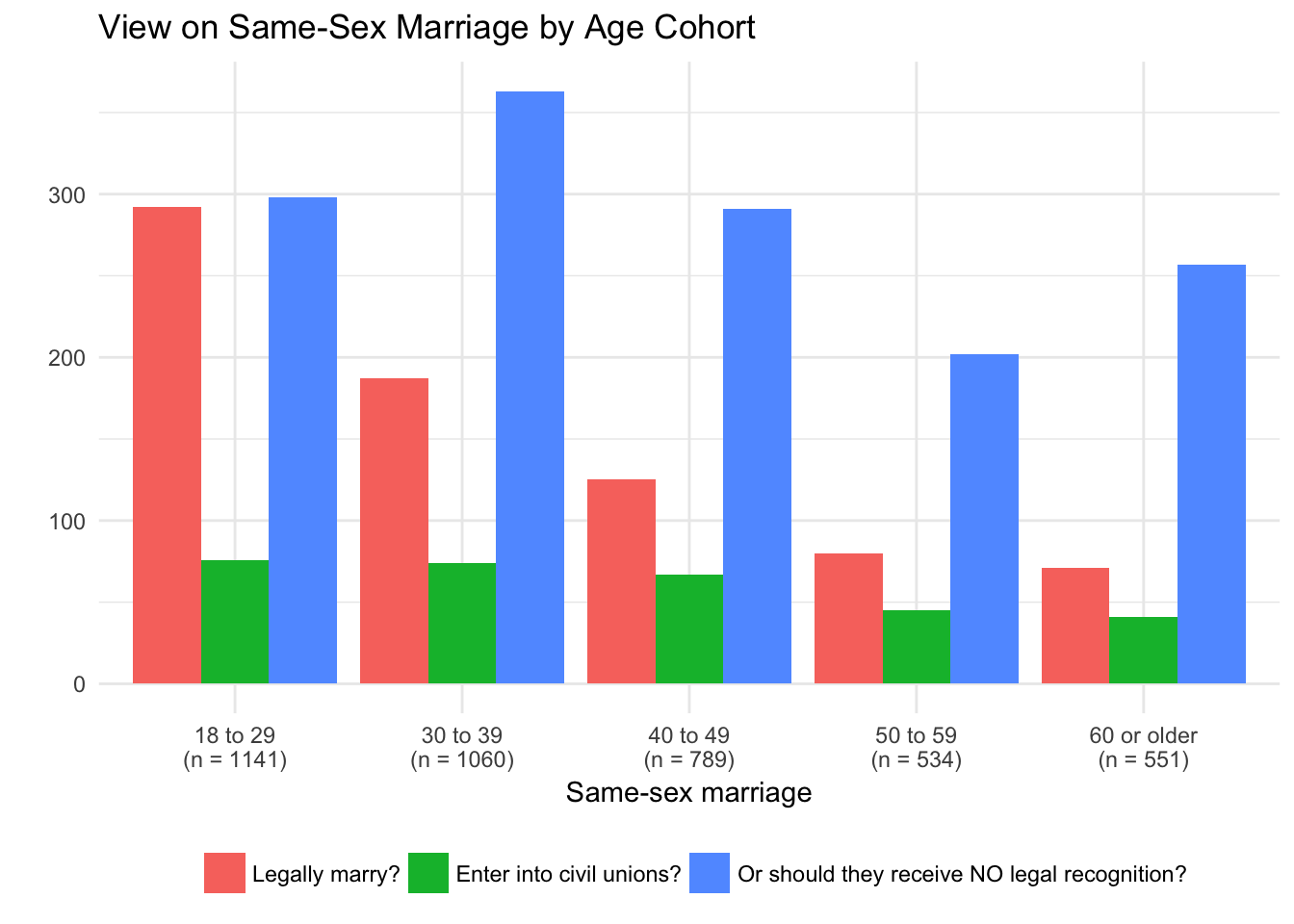And then, if you’re feeling particularly bananas, you can even plot three variables in this fashion. The only additional arguments you can use for this are the z_responses_to_ignore argument for any responses to the survey question used as your third variable you want to ignore, and the ncol argument, which you can set to 1 (ncol = 1) if you want the plots to print in one single column, or that you can ignore if you want them to print all on the same row. The latter is usually preferable visually.

plot_3_variables(
dataframe = dat,
x_variable = 'AGE_CAT',
y_variable = 'SAMESEX',
z_variable = 'BORNUS',
x_variable_label = 'Same-sex marriage',
y_variable_label = '',
x_axis_tick_labels = c('18-29', '30-39', '40-49', '50-59', '60+'),
bin_n = c(1141, 1060, 789, 534, 551),
legend_title = '',
plot_title = 'View on Same-Sex Marriage by Age Cohort by Where Born',
x_responses_to_ignore = NA,
y_responses_to_ignore = 'No opinion/NA')